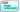Welcome To Basics In Maths

# CBSE 10th Class Maths Concept# This concept note is designed for CBSE 10th class maths students, This concept notes to do help students for the CBSE board examination and other competitive exams also…

## 1.REAL NUMBERS

Rational number: The number, which is written in the form of is called a rational number. It is denoted by Q.

Irrational number: – the number, which is not rational is called an irrational number. It is denoted by Q’ or S.

Prime number: – The number which has only two factors 1 and itself is called a prime number. (2, 3, 5, 7 …. Etc.)

Composite number: – the number which has more than two factors is called a composite number. (4, 6, 8, 9, 10… etc.)

Co-prime numbers: – Two numbers are said to be co-prime numbers, if they have no common factor except 1. [Ex: (1, 2), (3, 4), (4, 7) …etc.]

Euclid division lemma: – For any positive integers a and b, then q, rare integers exist uniquely satisfying the rules a = bq + r, 0 ≤ r < b.

To find H.C.F by using Euclid division lemma:

• For any two integers a and b (a > b).  Apply Euclid division lemma, to a and b, we find whole numbers q and r such that a = bq + r, 0≤r<b.
• If r = 0, b is the H.C.F of a and b. If r≠ 0, apply Euclid division lemma, to b and r.
• Continue the process till the remainder is zero. The divisor at this stage is the required H.C.F.

Note: –

• Euclid division lemma also called a division algorithm.
• Euclid division lemma is stated for only positive integers, it can be extended for all integers except 0.

The fundamental theorem of arithmetic:

Every composite number can be expressed as a product of primes, and this factorization is unique, apart from the order in which prime factors occur.

Ex: – 12 = 2 ×2× 3, 15 = 3× 5 and so on.

To find LCM and HCF by using prime factorization method:

H.C.F = product of the smallest power of each common prime factors of given numbers.

L.C.M = product of the greatest power of each prime factor of given numbers.

• ‘p’ is a prime number and ‘a’ is a positive integer, if p divides a2, then p divides a.
• Decimal numbers with the finite no. of digits is called terminating Decimal numbers with the infinite no. of digits is called non- terminating decimal. In a decimal, a digit or a sequence of digits in the decimal part keeps repeating itself infinitely. Such decimals are called non- terminating repeating decimals.

Decimal expansion of rational numbers:

Decimal expansion of rational numbers is either terminating or non-terminating repeating (recurring)decimals.

Ex: – 1.34, 2.345, 1.2222… and so on.

In p/q, if prime factorization of q is in the form 2m 5n, then p/q is terminating decimal. Otherwise non-terminating repeating decimal.

Decimal expansion of irrational numbers:

Decimal expansion of irrational numbers is non-terminating decimals.

Ex: – 1.414…., 1.314….

error: Content is protected !!